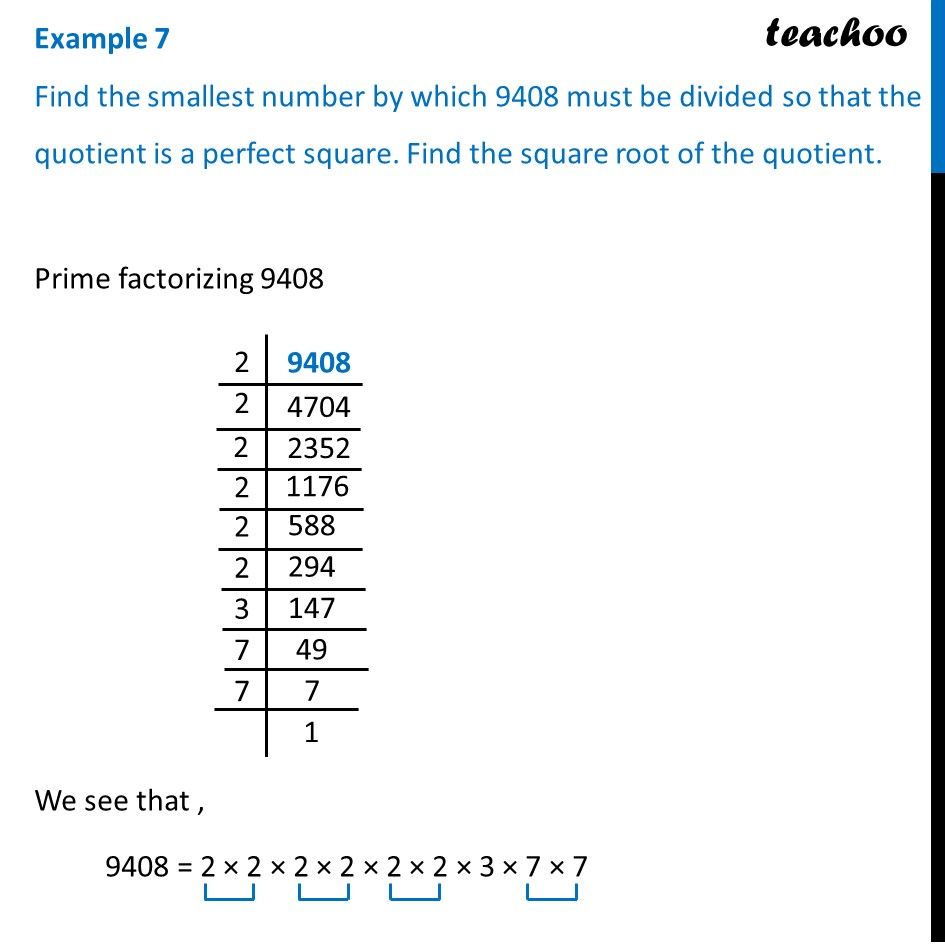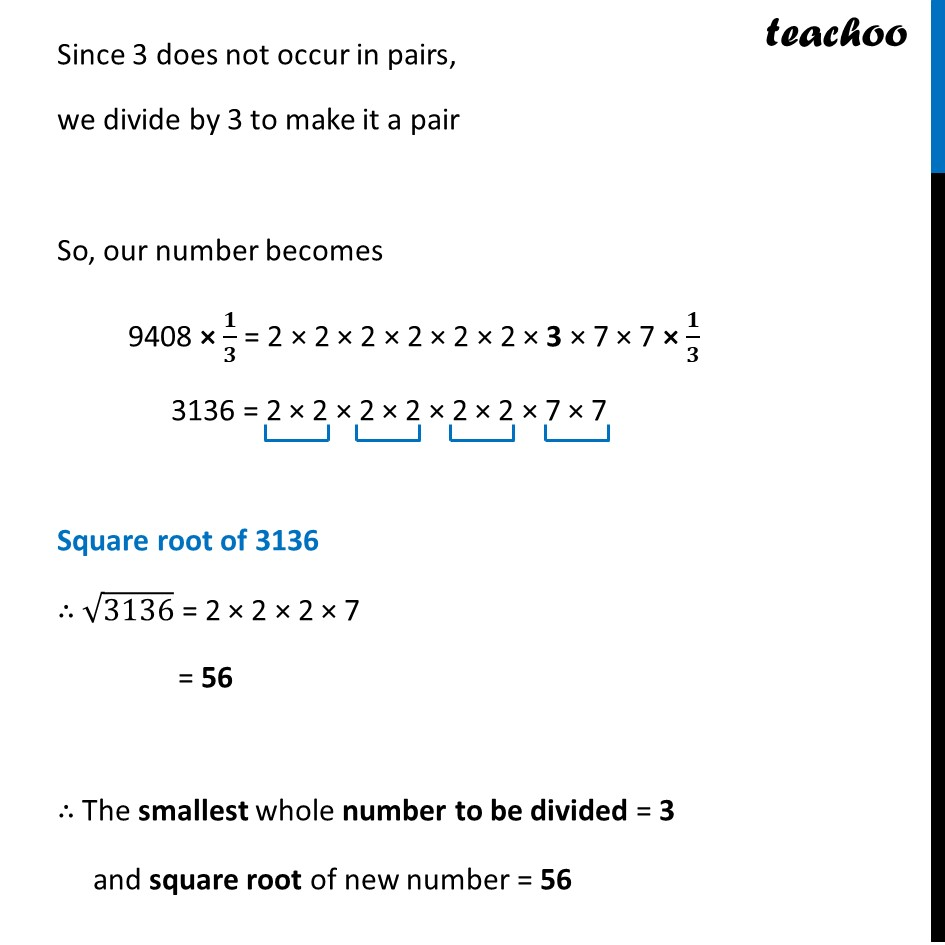Examples

Chapter 6 Class 8 Squares and Square Roots
Serial order wiseGet live Maths 1-on-1 Classs - Class 6 to 12

### Transcript

Example 7 Find the smallest number by which 9408 must be divided so that the quotient is a perfect square. Find the square root of the quotient. Prime factorizing 9408 Prime factorizing 9408 We see that , 9408 = 2 × 2 × 2 × 2 × 2 × 2 × 3 × 7 × 7 Since 3 does not occur in pairs, we divide by 3 to make it a pair So, our number becomes 9408 × 𝟏/𝟑 = 2 × 2 × 2 × 2 × 2 × 2 × 3 × 7 × 7 × 𝟏/𝟑 3136 = 2 × 2 × 2 × 2 × 2 × 2 × 7 × 7 Square root of 3136 ∴ √3136 = 2 × 2 × 2 × 7 = 56 ∴ The smallest whole number to be divided = 3 and square root of new number = 56